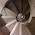## Monday, January 9, 2017

### Operations Before Numbers

Most elementary algebra books start on page one with a description of different sets of numbers that will be in use (naturals, integers, rationals, and reals). Then soon after they discuss the different operations to be performed on those numbers, the conventional order-of-operations, etc. This seems satisfying: you get the objects under discussion first, and then modifiers to be performed on those objects (nouns, then prepositions).

But the problem that's irked me for some time is this: the sets of numbers are themselves defined in terms of the operations. Most obvious is the fact that rationals are quotients of integers: a/b (b nonzero); so this presumes knowledge of division beforehand. Integers, too, are really differences of natural numbers (though usually expressed as something like "signed whole numbers"); they are fundamentally a result of subtraction. So in my courses I resolve this by coming out of the box on day one with a review of the different arithmetic operations, names of results, and their proper ordering; then on day two we can discuss the different sets of numbers thus generated.

Now, in other mathematical contexts  -- where you are only discussing one field at a time -- it is conventional to discuss the elements of a set first, and then the operations that we might apply on them second. That makes sense. But at the start of an elementary algebra course we tend to be cheating a bit by trying to consolidate a presentation of at least 4 different sets all at once. It would be fairly rigorous to present naturals and their operations (add, subtract, multiply, divide, etc.), and then integers (and their addition, subtraction, multiplication, etc.), then rationals and their operations (etc.), and then finally a separate discussion of real numbers and their operations (etc.). But that would take an inordinate amount of time, and the operations are so very similar that it would seem repetitive and wasteful to most of our students (outside of difference in closures, etc.).

So if the elementary algebra class wants to cheat in this fashion and present the whole menagerie of number categories in one lecture, I would argue that we need to abstract out the operations first, and then have those available to describe the differences in our sets of numbers second.

Thoughts? Are you still satisfied with describing numbers before operations?

#### 1 comment:

1.I totally agree, cuz must of the dificult part of math is the relation between numbers.

Great post